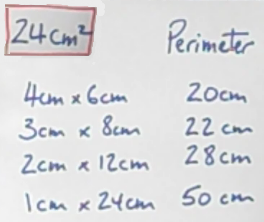#### You may also like### Framed

Seven small rectangular pictures have one inch wide frames. The frames are removed and the pictures are fitted together like a jigsaw to make a rectangle of length 12 inches. Find the dimensions of the pictures.### Tilted Squares

It's easy to work out the areas of most squares that we meet, but what if they were tilted?### Four or Five

The diagram shows a large rectangle composed of 9 smaller rectangles. If each of these rectangles has integer sides, what could the area of the large rectangle be?

# Perimeter Possibilities

### Why do this problem?

Many mathematical tasks about area and perimeter focus on the two separately. This problem combines both by inviting students to consider the different possibilities for the perimeter when the area of a rectangle is fixed.

The problem offers opportunities to gain insights that can be applied to a variety of other area and perimeter tasks such as Can They Be Equal?, Changing Areas, Changing Perimeters, Warmsnug Double Glazing, and Fence It.

### Possible approach

This printable worksheet may be useful: Perimeter Possibilities.

This problem can lead to many different questions that can be explored in different ways to suit your class. You may wish to watch the two videos in the problem and then introduce the task in a similar way:
"I'm thinking of a rectangle with an area of $24$cm$^2$. What could its perimeter be?"
Students could write their responses on mini-whiteboards. Then, once the class have had time to come up with their answer(s), gather responses on the board in a table like this one:"Look at the dimensions and perimeters. What do you notice?"
Write on the board any 'noticings' that the students come up with. This might include:

All the perimeters are even
The perimeters are getting larger
The sum of the dimensions is equal to half the perimeter
The dimensions are all factor pairs of $24$
As the perimeter gets larger the rectangle gets 'narrower'/'skinnier'/'thinner'.

"It would be nice to be able to explain what you have noticed. Take a couple of minutes to think by yourself and then talk with your partner to see what explanations you can come up with. Will they be true for rectangles with other areas?"

After some discussion: "Actually, the rectangle I was thinking of had an area of $24$cm$^2$ and a perimeter of $35$cm. Can you use your insights to work out the dimensions of my rectangle?" Allow time for a proper discussion about developing a strategy to solve the problem using the students' initial insights.

"In pairs, I would like each of you to think of a rectangle, work out its area and perimeter, and then give this information to your partner to see if they can work out the dimensions of your rectangle.
Do this a few times and keep a record of your rectangles and the methods you are using.

This is a really good opportunity for students to use trial and improvement. Although there are algebraic methods, trial and improvement allows students to use their insights to get to the answer quite quickly and efficiently.

The lesson could be rounded off by offering students a challenge such as "I'm thinking of a rectangle whose perimeter is $21$ and whose area is $20$. What are the dimensions of my rectangle?"

### Key questions

Are the perimeters of rectangles always even?
Why do the rectangles get thinner as the perimeter gets larger? (for a fixed area)

### Possible support

Students could be asked to consider rectangles with a fixed perimeter before moving on to rectangles with a fixed area.

### Possible extension

Here are some possible follow-up questions:

• What other odd number perimeters can you make, if the area is $24$cm$^2$?
• What is the smallest perimeter you can make, if the area is $24$cm$^2$? What about the largest perimeter?
• Which perimeters in between is it possible to make?

More generally...

• Is it possible to make a rectangle with a fractional perimeter but a whole number area?
• Is it possible to make a rectangle with a whole number perimeter but a fractional area?

Can They Be Equal?, Changing Areas, Changing Perimeters, Warmsnug Double Glazing, and Fence It could be tackled next.